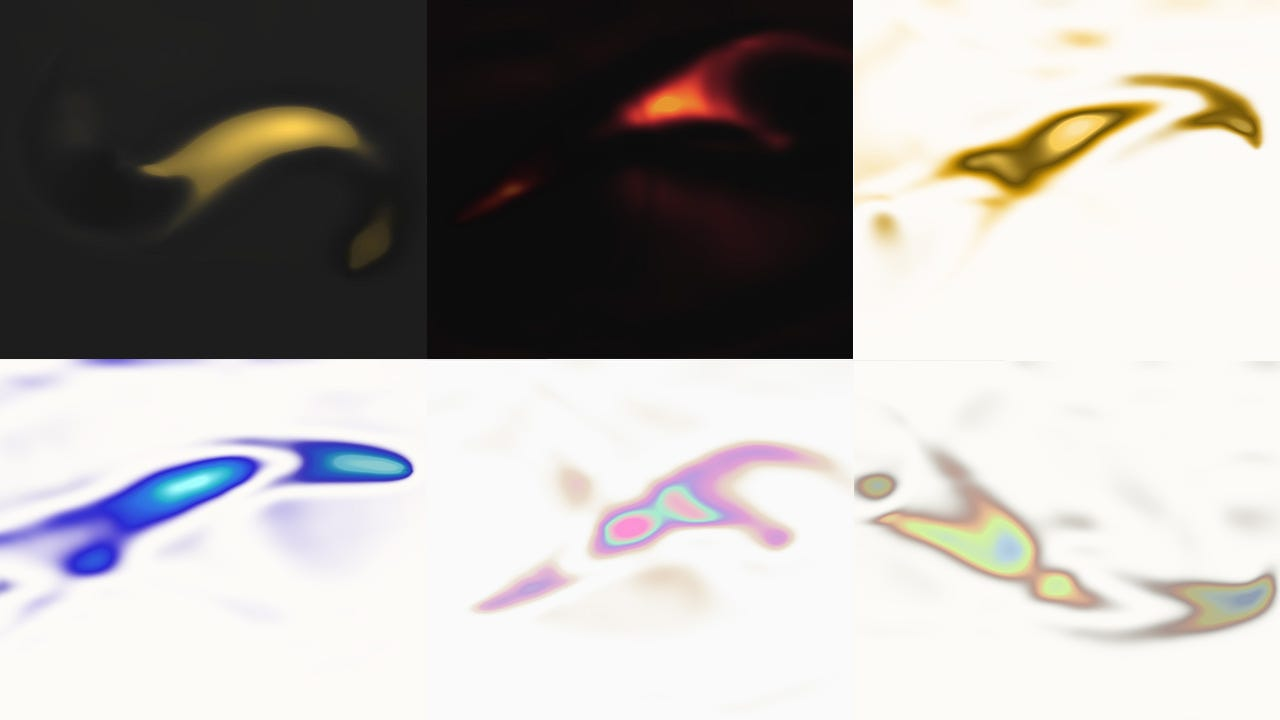# The fluid effectSome of the different tests I’ve made while trying to find the result that would suit our needs.
`// ligths & shadowsfloat lightStrength = 5.0;float lights = max(0.001, ripples.r - (0.7 + lightStrength * 0.025));float shadow = max(0.001, 1.0 - (ripples.r + 0.5));// get values to mix white and the colors for lights// decrease lights area by multiplicating it with a float < 1// then apply a pow() operation to sharpen the area// finally multiply back the result with a big float number to increase the effect strengthfloat lightMixValue = clamp(pow(lights, 3.5) * 5.0, 0.0, 1.0);float lightMixValue2 = clamp(pow(lights * 0.95, 5.0) * 10.0, 0.0, 1.0);float lightMixValue3 = clamp(pow(lights * 0.9, 6.5) * 15.0, 0.0, 1.0);float lightMixValue4 = clamp(pow(lights * 0.85, 7.0) * 30.0, 0.0, 1.0);float lightMixValue5 = clamp(pow(lights * 0.8, 7.25) * 70.0, 0.0, 1.0);float lightMixValue6 = clamp(pow(lights * 0.75, 8.5) * 100.0, 0.0, 1.0);float lightMixValue7 = clamp(pow(lights * 0.7, 10.0) * 50.0, 0.0, 1.0);// apply colors to lights// [fluid1 ... fluid7] are predefined vec3 colorsvec3 lightColor = mix(color.rgb, fluid1, lightMixValue);lightColor = mix(lightColor, fluid2, lightMixValue2);lightColor = mix(lightColor, fluid3, lightMixValue3);lightColor = mix(lightColor, fluid4, lightMixValue4);lightColor = mix(lightColor, fluid5, lightMixValue5);lightColor = mix(lightColor, fluid6, lightMixValue6);lightColor = mix(lightColor, fluid7, lightMixValue7);// repeat for shadowsvec3 lightColor = mix(color.rgb, fluid1, lightMixValue);lightColor = mix(lightColor, fluid2, lightMixValue2);lightColor = mix(lightColor, fluid3, lightMixValue3);lightColor = mix(lightColor, fluid4, lightMixValue4);lightColor = mix(lightColor, fluid5, lightMixValue5);lightColor = mix(lightColor, fluid6, lightMixValue6);lightColor = mix(lightColor, fluid7, lightMixValue7);`